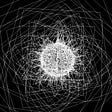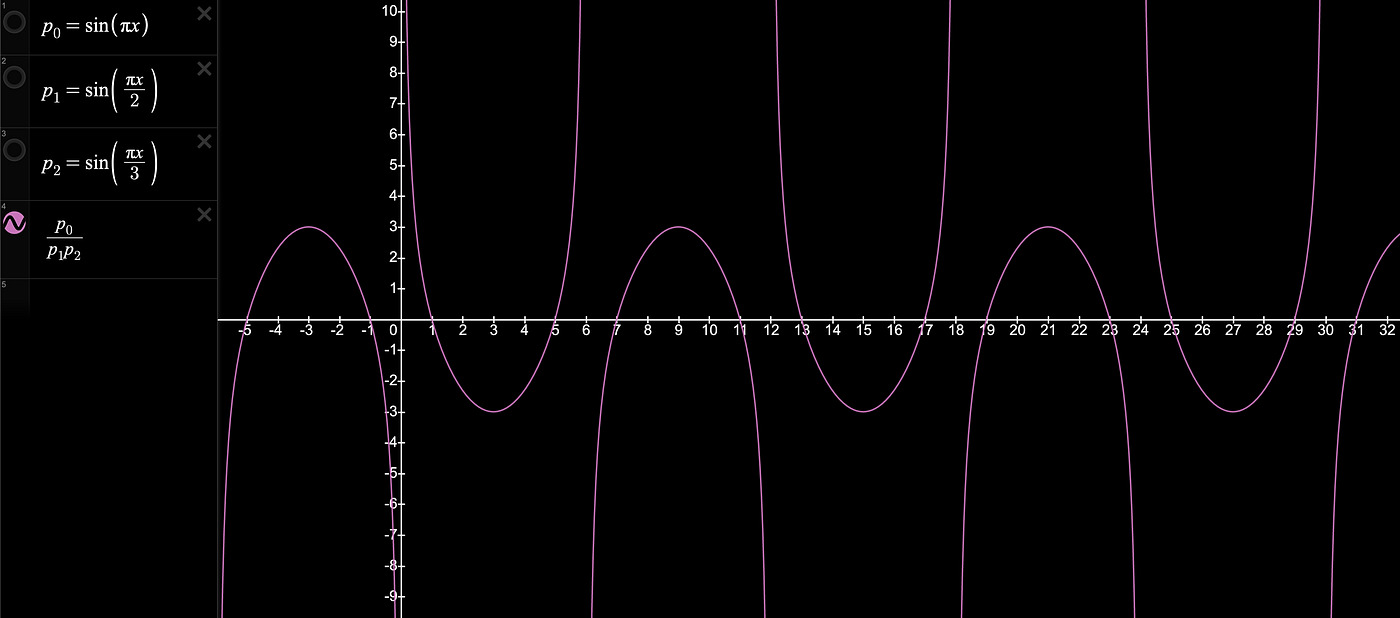A Comparison of the Waves Functions of Prime Numbers 2 & 3 Against the Wave Function of All Integers.

# The Prime Equation

## Primal Wave Interference

Where:Pa Represents a Wave that Passes through Every Prime Number & Their Multiples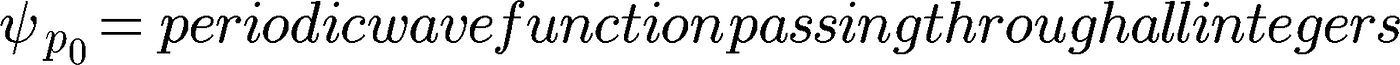Periodic Sine Wave Function Passing Through All Integers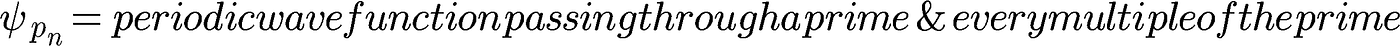Periodic Sine Wave Function Passing Through a Prime & Every Multiple of the Prime

Expanded form:

# Solve for 0:

Wave function for all integers:

(Observe that the wave passes through every integer at y = 0)

Wave function of p₁ (i.e. the prime number 2):

(Observe that the wave passes through every other integer at y = 0, that is every even number 2, 4, 6…)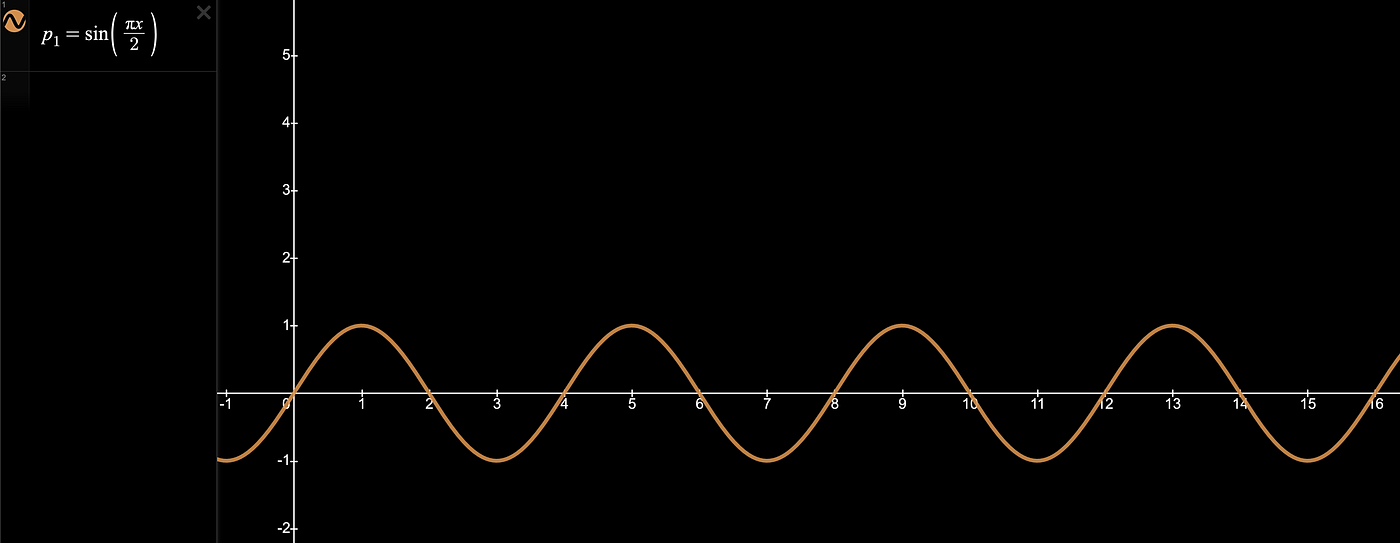Periodic Wave Function Passing Through The Prime Number 2 & Every Multiple of Prime Number 2

The interference pattern for both wave functions shows that both waves meet at y=0 only at even numbers:

Now that we have defined a waveform that passes through all integers and a waveform passing through the prime number 2 and all multiples of the prime number 2, we can compare the interference pattern of the two waves by solving for zero. Simply dividing p₀ by p₁ (p₀/p₁). The solution of the expression (the vertical purple lines) shows that all even numbers can be expressed through the wave function of p₁, which is the wave function for the prime number 2 and every multiple of the prime number two, that is 4, 6, 8, 10,…,34, etc.:

Solving for 0, it is evident only odd numbers are left to be expressed as a wave function and are spaced every other number — which is has been observed as the Twin Prime Conjecture. The first prime wave function (p₁) allows for 50% of all integers to be expressed and what’s left are all the integers where no wave function passes 1, 3, 5, 7, 9, 11 — that is every odd number.

Once the equation is solved for p₁, we see that the twin prime conjecture is nothing more than the observation that after solving for P₁, all the even numbers are expressible, and only the odd numbers remain as possible primes, and we know that odd numbers are only one number apart a.k.a. “twins.”

Therefore, it can be deduced that there are an infinite number of twin primes possible.

N.B. — This does not prove with 100% certainty, that there are an infinite number of twin primes (although this certainly appears to be the case). It only shows that there are infinite number of twin primes POSSIBLE. The only thing that can be deduced with 100% certainty is that there are infinite possible locations for twin primes to show up — thus it is no surprise that twin primes exist.

# Solving for p₂:

Once the function is solved for p₁, the next step is to solve p₂, where the function equals 0 for the waves of all integers and the wave of p₂.

Integrating the wave expression of p₂ into the function, it is seen that not only is there now an expression for 3, but also for 9 and 15, etc., ad infinitum.

N.B. — Because odd number products alternate between odd and even numbers, and only even numbers currently have a wave expression, we can deduce that p₂ (i.e. the prime number 3) will be a solution for every other number, solving for 3, not 6, 9, not 12, 15, not 18, 21, not 24, ad infinitum.

By removing the function we can see an expression of perfect infinite symmetry in the Prime Equation of p₀/(p₁*p₂):

# Solving for p₃:

From the result above, it’s clear the next prime is the number 5.

Thus a new wave to express p₃ is required.

N.B. — zooming out, it is shown that the wave of p₃ is not only an expression for 5, but also for 25, 35, 55, and all other wave expressions that end in 5, ad infinitum. Thus once the wave expression for p₃ is introduced, there are no prime numbers that end in 5.

Inserting the wave expression for p₃ into the formula creates the following result:

# Solving for p₄:

From the result above, it’s clear the next prime is the number 7.

Thus a new wave to express the fourth prime i.e. p₄ is required.

# Conclusion:

Primes aren’t random. They’re exactly where they are needed to express a quantity that cannot be expressed with any previous prime.

Finding the location of any prime is contingent on solving the dynamic prime equation, where each successive wave expression in the equation, is not only an expression for the next 0 of the function but also for an infinite number of 0s on the y-axis further down the series.

Using this equation, it can be seen that the wave patterns of the prime numbers (prime building blocks) overlap and interference with each other — and it quickly becomes apparent that a prime can only end in 1, 3, 7, 9.

The key is to understand that it cannot be known for certain which results are primes until the equation has been resolved for all previous primes up to the number being evaluated.

# Bonus 1:

The last statement of the conclusion isn’t 100% accurate.

For any number “x” evaluated, it can be known to be a prime with 100% certainty as soon as the function has been run for all numbers ≤ x/2 — that is to say, just over half the number “x” being evaluated.

Another way of stating this is to say that:

Once the equation is solved for up to P to the nth prime, it is certain that all 0 results of the function greater than P to the nth prime and less than twice the product of P to the nth prime are also prime.

This is because, if the equation has been solved for up to P to the nth prime, it is 100% certain that there are no wave expressions greater than P to the nth prime and less than twice the product of P to the nth prime that exist to express the waves where the function is 0.

e.g.

• p₃ is the wave expression for the prime number 5
• 2*p₃ is the wave expression for the composite number 10
• The function of Pₐ=0 at x=7
• We know that every wave expression <p₃ already exists and any wave expression >p₃ will result in a number greater than 7
• Therefore, it can be known with 100% certainty that 7 is a prime.

# Build Your Own Equation Expressed as a Dynamic Prime Wave:

N.B. — If you find any errata in the above — please comment for me to correct.

After further research I have encountered a formula that appears to be a variation of the function I have presented which is the Prime Zeta Function a.k.a. Euler’s Product Formula developed by Leonard Euler.

# Bonus 2:

Here’s the start of a Python program that shows the results of the equation above by running the functions for Primes 1–5, i.e. 2, 3, 5, 7, & 11.

As you can see each function for the next prime contains all previous equations to compare against.

`# <<< imported python libraries/commands >>>import math# <<< program functions >>># <<< variables >>>pi =round(2*(math.acos(0.0)), 10)# <<< Comparing wave expression for all integers against wave expression for prime numbers >>>def p1():    x=1    while x >= 1:        x += 1        f0 = round(math.sin(pi*x))        f1 = round(math.sin((pi*x)/2))                if f0 == (f1):            return(round(x))def p2():    x=1    while x >= 1:        x += 1f0 = round(math.sin(pi*x))        f1 = round(math.sin((pi*x)/p1()))                if f0 != (f1):            return(round(x))def p3():    x=1    while x >= 1:        x += 1                f0 = round(math.sin(pi*x))        f1 = round(math.sin((pi*x)/p1()))        f2 = round(math.sin((pi*x)/p2()))                if f0 != (f1 * f2):            return(round(x))def p4():    x=1    while x >= 1:        x += 1                f0 = round(math.sin(pi*x))        f1 = round(math.sin((pi*x)/p1()))        f2 = round(math.sin((pi*x)/p2()))        f3 = round(math.sin((pi*x)/p3()))                if f0 != (f1 * f2 * f3):            return(round(x))def p5():    x=1    while x >= 1:        x += 1                f0 = round(math.sin(pi*x))        f1 = round(math.sin((pi*x)/p1()))        f2 = round(math.sin((pi*x)/p2()))        f3 = round(math.sin((pi*x)/p3()))        f4 = round(math.sin((pi*x)/p4()))                if f0 != (f1 * f2 * f3 * f4):            return(round(x))`

--

--

--

## More from DK

Human Being, b. circa 1990 ~ planet Terra, Via Lactea Galaxia

Love podcasts or audiobooks? Learn on the go with our new app.

## A Probability Challenge## Generalized competition problems | Part 4— A number theory problem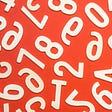## Your Life is Too Complicated.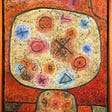## Basic Set Theory by Exercises: First Order Logic — Propositional Logic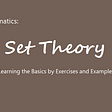## When you’ll be surprised next time?## DK

Human Being, b. circa 1990 ~ planet Terra, Via Lactea Galaxia

## Intro to Graph Theory## Five free resources to get you started with the Lean Theorem Prover## Just what are numbers, really? (Part I)## Animated Geometric Pattern Using Python